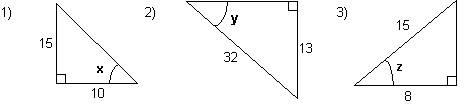# Calculating angles using trigonometric ratios - Tutorial

math tutorials > calculating angles of a triangle using trig ratios

## The size of an unknown angle in a right-angled triangle can be calculated using the trigonometric ratios.

What you will need:

You will need to use the INV / Shift / 2ndf key on your scientific calculator.

## Steps

1. Label the sides.
2. Identify which trig. ratio uses these sides.
3. Calculate the angle.

e.g.## Trigonometry problems for you to try.